×#### Thank you for registering.

One of our academic counsellors will contact you within 1 working day.

Click to Chat

1800-1023-196

+91-120-4616500

CART 0

• 0

MY CART (5)

Use Coupon: CART20 and get 20% off on all online Study Material

ITEM
DETAILS
MRP
DISCOUNT
FINAL PRICE
Total Price: Rs.

There are no items in this cart.
Continue Shopping• Complete JEE Main/Advanced Course and Test Series
• OFFERED PRICE: Rs. 15,900
• View Details

```Constructions Exercise 17.3

Question: 1

Draw △ABC in which AB = 3 cm, BC = 5 cm and ∠Q = 70°.

Solution: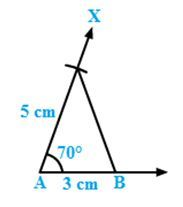Steps of construction:

Draw a line segment AB of length 3 cm.

Draw ∠XBA=70°.

Cut an arc on BX at a distance of 5 cm at C.

Join AC to get the required triangle.

Question: 2

Draw △ABC  in which ∠A=70°., AB = 4 cm and AC= 6 cm. Measure BC.

Solution: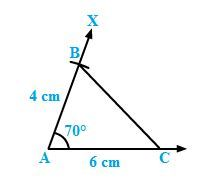Steps of construction:

Draw a line segment AC of length 6 cm.

Draw ∠XAC=70°.

Cut an arc on AX at a distance of 4 cm at B.

Join BC to get the desired triangle.

We see that BC = 6 cm.

Question: 3

Draw an isosceles triangle in which each of the equal sides is of length 3 cm and the angle between them is 45°.

Solution: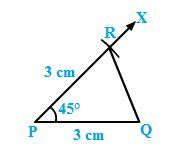Steps of construction:

Draw a line segment PQ of length 3 cm.

Draw ∠QPX=45°.

Cut an arc on PX at a distance of 3 cm at R.

Join QR to get the required triangle.

Question: 4

Draw △ABC  in which ∠A = 120°, AB = AC = 3 cm. Measure ∠B and ∠C.

Solution: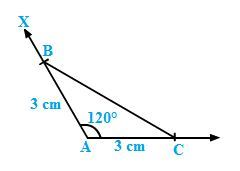Steps of construction:

Draw a line segment AC of length 3 cm.

Draw ∠XAC = 120°.

Cut an arc on AX at a distance of 3 cm at B.

Join BC to get the required triangle.

By measuring, we get ∠B = ∠C = 30°.

Question: 5

Draw △ABC  in which ∠C = 90° and AC = BC = 4 cm.

Solution: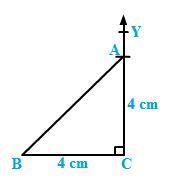Steps of construction:

Draw a line segment BC of length 4 cm.

At C, draw ∠BCY=90°.

Cut an arc on CY at a distance of 4 cm at A.

Join AB. ABC is the required triangle.

Question: 6

Draw a triangle ABC in which BC = 4 cm, AB = 3 cm and ∠B = 45°. Also, draw a perpendicular from A on BC.

Solution: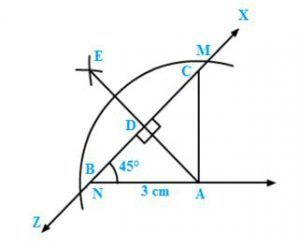Steps of construction:

Draw a line segment AB of length 3 cm.

Draw an angle of 45° and cut an arc at this angle at a radius of 4 cm at C.

Join AC to get the required triangle.

With A as centre, draw intersecting arcs at M and N.

With centre M and radius more than half of MN, cut an arc on the opposite side of A.

With N as centre and radius the same as in the previous step, cut an arc intersecting the previous arc at E.

Join AE, it meets BC at D, then AE is the required perpendicular.

Question: 7

Draw a triangle ABC with AB = 3 cm, BC = 4 cm and ∠B = 60°. Also, draw the bisector of angles C and A of the triangle, meeting in a point O. Measure ∠COA.

Solution:Steps of construction:

Draw a line segment BC = 4 cm.

Draw ∠CBX = 60°.

Draw an arc on BX at a radius of 3 cm cutting BX at A.

Join AC to get the required triangle.

Angle bisector for angle A:

With A as centre, cut arcs of the same radius cutting AB and AC at P and Q, respectively.

From P and Q cut arcs of same radius intersecting at R.

Join AR to get the angle bisector of angle A.

Angle bisector for angle C:

With A as centre, cut arcs of the same radius cutting CB and CA at M and N, respectively.

From M and N, cut arcs of the same radius intersecting at T

Join CT to get the angle bisector of angle C.

Mark the point of intersection of CT and AR as 0.

Angle ∠COA = 120°.
```### Course Features

• 728 Video Lectures
• Revision Notes
• Previous Year Papers
• Mind Map
• Study Planner
• NCERT Solutions
• Discussion Forum
• Test paper with Video Solution